## How to make speech bubbles draw themselves: a story of pain (part 3)

In the part two of this saga, we’ve discovered that not using brute force is not gonna be an option. After our first attempt at brute force failed miserably, we’ll have to find another way.

# Me orc, me smash: Brute force revisited

Upon review, it turns out that our first solution was actually kinda close to working. Our algorithm kinda looked like this:

1. Find the center of the ellipse
2. Find aspect ratio of the text
3. Assume that the aspect ratio we found is the same as the aspect ratio of our ellipse
4. Squash text into the square and draw a circle around it
5. Calculate the radius of the circle using maths
6. Unsquash the circle, get ellipse (or two radii that define it)

The obvious problems with this approach were:

• assumption under point #3 is incorrect
• this means the radius we calculated was also wrong, and often too tight

We can work around that. We only really need to add very little on top of what we already have:

1. Calculate radius of the circle using maths
2. **Increase that radius by some factor in order to give us some breathing room**
3. Unsquash the circle, get ellipse (or two radii that define it)
4. **Try to make ellipse smaller, one small step at a time**
5. Repeat #8 a few times, use the best result you get

Steps 8/9 can be done using a kind of binary search, and it goes roughly like this:

1. Calculate whether all points are in the ellipse for given radii.
2. If all points are inside the ellipse, decrease radii for half of a step
3. If any of the points are outside the ellipse, increase radii for half of a step
4. Decrease step by half
5. If step is sufficiently small, you can stop
6. If step isn’t sufficiently small, go back to #1

In our case, we have two ‘steps’ (each for one of the radii). Before starting our algorith, we determine two values for each of the radii:

• “Radius can’t be bigger than this” number (e.g. width of a given line of text)
• “Radius can’t be smaller than this” number (e.g. half of the width of a given line of text)

Step is the difference between the two (different for each radius). We use the bigger of the two numbers as our radius.

This works great, but we can improve it even further. Inside every step, we can try decreasing size of one radius while keeping the other radius the same. This can help us find smaller, better-fitting ellipses at the expense of some extra calculations (to the tune of O(n²)).

# Trying it out

Now that we wrote the code, it’s time to try it out. Results ended up being slightly disappointing: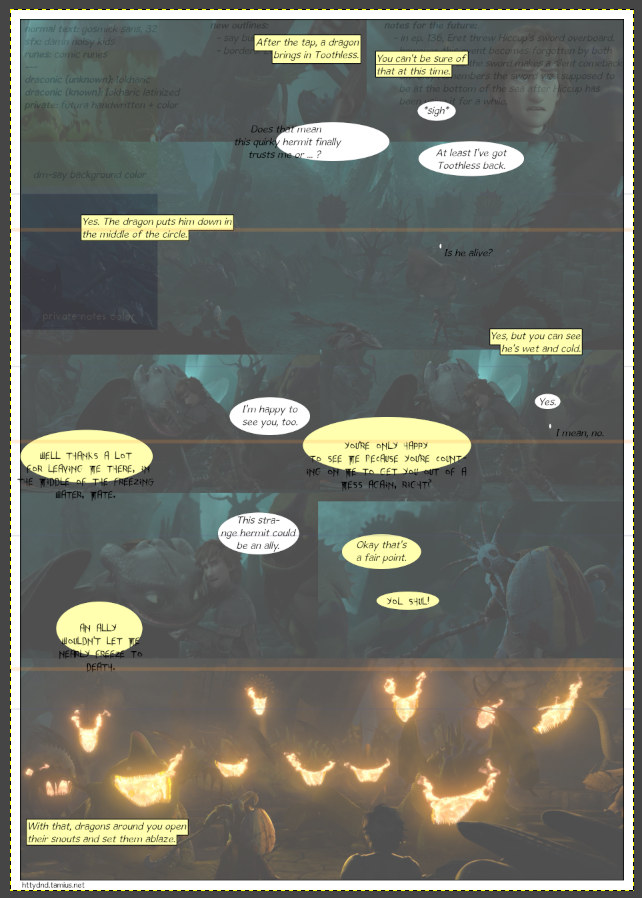Yikes. That’s worse than our previous test. We even managed to fail to draw a rectangle properly.

What is worse: there doesn’t seem to be neither reason nor rhyme to why things don’t work. Our brute force approach was used at least five times and has two failures (where bubble is way too small). Multiline texts — the ones that very probably don’t use the bruteforce approach at all — are often off-center and sometimes even too small (all off-center bubbles are too small, so text doesn’t fit in the ellipse):

# What gives?

Upon closer examination, it turns out that some ellipses that should be drawn with brute force method weren’t drawn by brute-force method. Secondly, despite determining ellipse’s radius by brute force, we still always use our matrix to determine one or both center coordinates. Our ‘high maths’ approach is giving us slightly suspect — and possibly even wrong — results.

The one thing to consider when writing code is that computers are generally fairly terrible with very tiny (and very big) numbers. The tinier your number (we’re talking anything past fifth decimal), the less “accurate” it is. The values the matrix calculation spat out contained some very tiny numbers. When those numbers were used to perform further calculations, the errors grew and we got garbage results.

Let’s forget the ‘high maths’ approach for a while and try to brute force everything.

# Giving brute force another try

## Step 1: determining the center of the ellipse

This one is going to be easy. We still only work with 4 points at the time. To get the ‘center point’, we find the average of the four coordinates. Then, we try to find the intersection between the lines connecting the points at the opposite sides of the quadrangle the four points form. If the point of intersection is mirrored over the ‘center point,’ it gives us the center of our ellipse.

## Step 2: apply smash, remove smart

We feed the center of the ellipse we got from step 1 into the brute force algorithm we discussed earlier, run some test and …

Good news is: brute force does find better results than attempt at using high maths. No bubble is drawn off-center, all bubbles are correctly sized. The reason for the crash is a weird point selection: one of the diagonals (in at least one of the 4-point subsets of the last bubble) is vertical. In order to calculate the center of our to-be-ellipse, we need to know the slope of thes line between the opposing sides. There’s a bit of a problem though: if you’re trying to calculate the slope of a vertical line in a coordinate system, you’re going to faceplant into a division by zero.

Computers don’t like dividng by zero.

We can quickly see another thing: if we try to determine the center for our ellipse using these four points, we’ll just get less-than-optimal solution. That means we can skip it — all it would do is waste our time.

Let’s see if that fixed our probem: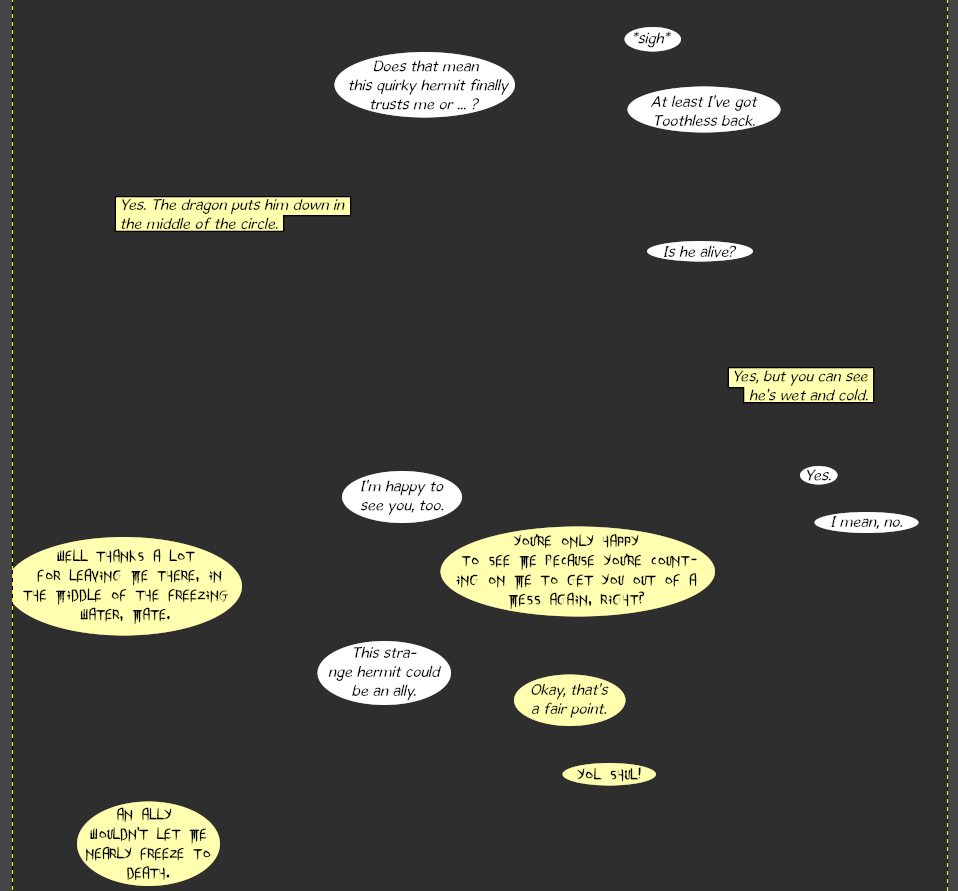And all is right. As far as performance goes, “stopwatch test” says brute-force approach isn’t really that much slower than the “proper”, “high maths” approach.

# This leads us to the conclusion:

Lots of people love the “work smart, not hard” approach to things. Sure, using a little bit of brainpower to avoid using a lot of force is nice. But let’s not forget that sometimes, the opposite applies: a little bit of brute force can save us from using lots of brainpower.

Moral of the story: don’t overthink your programs, I guess? Sometimes, simple is better and ‘approximate’ is good enough.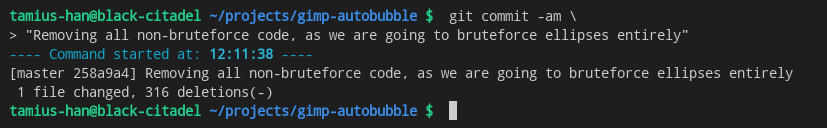You’re looking at one or two weeks of my free time going down the drain. Just like that.

## How to make speech bubbles draw themselves: a story of pain (part 2)

When we left off last time, I still thought this wasn’t going to be that bad. I was wrong.

So we’re back to square one and I’m looking at the first answer about my problem that I’ve found over at math stackexchange. When I first saw it, I thought I haven’t watched enough Rick&Morty to understand it, but after playing around with it for way longer than I should have I started figuring shit out.

If you plop 4 points into the following equation:

ax² + by² + cx + dy + e = 0

And solve the system of those four equations, you should notice that the variables ‘a’, ‘b’, ‘c’ and ‘d’ are all some fraction or multiplier of ‘e’. Even without knowing the value ‘e’, we can calculate the center of the ellipsis. If we want radius, we need to know ‘e’. Assuming ‘e’ is -1 seems to do the job the way we wanted, though.

Since our convex hull often contains more than 4 points, we try to calculate the ellipse for every combination of 4 points (out of all points on the hull). Note that because we’re lazy, we don’t calculate the hull. We just take upper two corners from each row in the upper half of text and the bottom two corners from each row in the bottom half of text. If the text has odd number of rows, we include all four corners in the convex hull.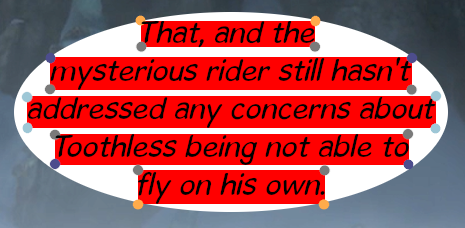All edge points are marked with a circle.⬤ — point not on the ‘convex hull’, therefore we ignore it.⬤⬤⬤ — Points we assume are on the convex hull. No biggie if they aren’t as we throw out garbage results later

We calculate radii for each combination of 4 points that we have. We check if the new ellipse contains all the points of the hull (either inside or on the edge). We reject the result if it doesn’t. If we don’t reject the result, we compare it with the last result we didn’t reject. If the ellipse is bigger than the last result, we reject it. If not, this is our new result. Repeat until you’ve ran out of combinations.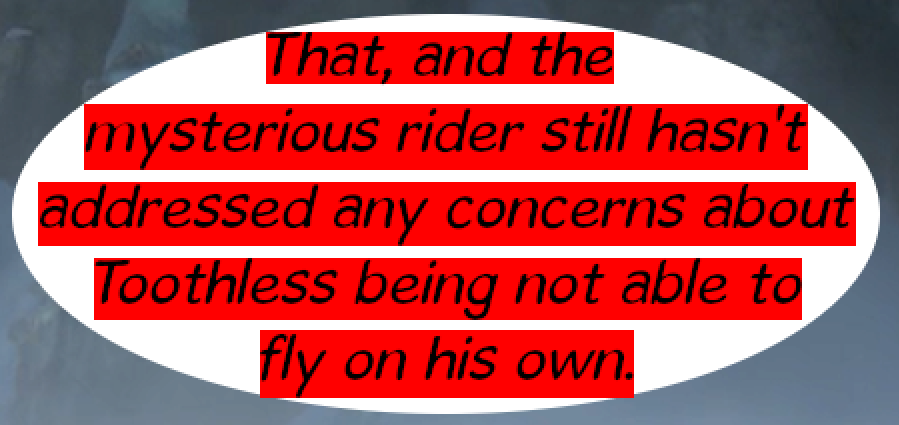It fits, more or less. There appears to be some rounding/off-by-one error down there at the bottom, but nothing that’s terribly problematic.

Sooner or later, though, we run into a problem. What if ‘c’ and ‘d’ are free variables instead of ‘e’? This happens if any of the four points are horizontally or vertically symmetrical relatively to the center of the ellipse. For example, if you plug points 0,0; 4,0; 0,2; 4,2 into the equation, you’ll find that ‘a’ is some fraction of ‘c’ and ‘b’ is some fraction of ‘d’. You can still calculate center from that, but you can’t get the radii. Even worse is the case of 0,2; 2,0; 4,0; 6,2: symmetry across only one axis means you can only get one coordinate of the center because there’s infinitely many solutions.

Since we’re after only one specific solution, for us ‘infinitely many solutions’ equals ‘no solution.

### Forsaken cheats

In the second example from above, we could do some additional maths in order to find the center. We’re only interested in the smallest possible ellipse, so we could calculate some limits in order to get the other center coordinate. But word on the streets is that cheating is easier and could get us results that are close enough to what we want.

Turns out breaking symmetry isn’t too hard — we just need to ensure that points in the left and right halves don’t share the same ‘y’ coordinate, and points in top and bottom halves don’t share the same ‘x’ coordinate.

The first bit of this task can be easily achieved: since every row has two points with same ‘y’ coordinate (and since ‘y’ coordinate can’t repeat across multiple rows), we just need to shift one point a bit up and the other a bit down (or keep it at the same place).

Shifting ‘x’ coordinates is a bit more problematic: two different rows can absolutely contain same ‘x’ coordinate — and what is worse, if we shift the ‘x’ coordinate in any way, we may create the situation we’re trying to avoid. However, we know the following things:

• point coordinates are whole numbers only (We get points by reading pixels, and there’s no such thing as ‘half a pixel’)
• only points in the opposite rows are required to have different x and y coordinates⬤ — point not on the ‘convex hull’, therefore we ignore it.⬤⬤⬤ — Points of the same color aren’t allowed to share any coordinate.

If two neighbouring rows (e.g. top two rows in text with more than 3 rows) have points that share the same ‘x’ coordinate, it doesn’t really matter because ellipse containing those points would be rejected for being too small anyway.

This allows us to easily apply required offsets:
* upper left: x -= 0.5, y -= 0.5
* upper right: x -= 0.5
* bottom-left: y += 0.5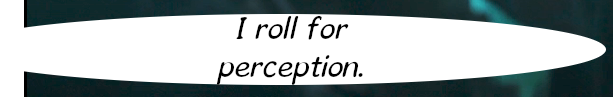So I thought I could do it by shifting points a little outward. Turns out that two-line and one-line rows disagree. At the end of the day, “losing” half pixel of space won’t really be noticed when bubble is more than hundred pixels across most of the time — and when it’s not, the radius is still in high double digits. So let’s change that a bit:

• upper left: x -= 0.5, y -= 0.5
• upper right: x -= 0.5
• bottom-left: y += 0.5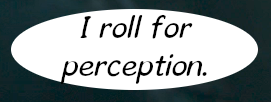Not quite what we want, but better than what we had before.

Let’s try that out a bit more: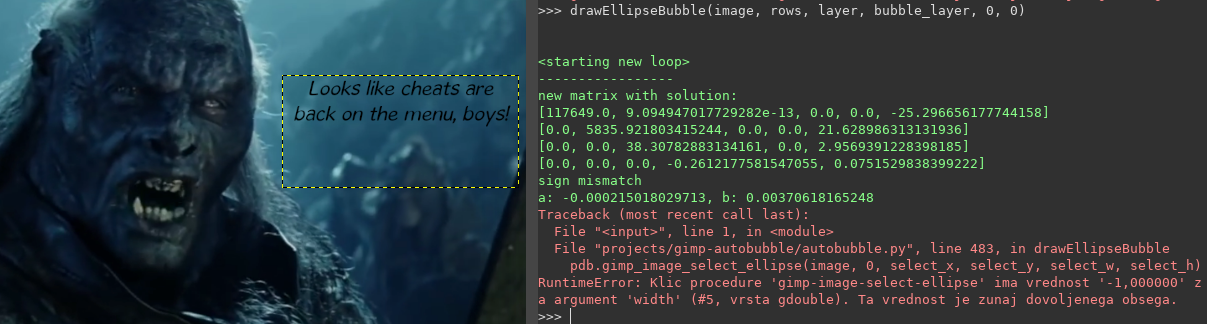Oopsie whoopsie. Fucky wucky.

Turns out I spoke too soon.

### The Right Way™

Sometimes, we don’t have enough data to determine where the center is. Sometimes, we will only get enough data to determine one of the center coordinates. For example, if you only have four points to go off, and if said points would form a symmetrical (acute) trapezoid they were to be connected with lines — and since our text is centrally aligned, that happens almost every time we want to draw a bubble around two lines of text — you can still determine horizontal center.

Turns out this comes out handy: we can determine one of the coordinates using the kind of “cheating” approach we used before, but with some twists:

• We split points in two groups: those to the left and those to the right of the horizontal center (top and bottom if we have the vertical center of the ellipse)
• Find the vertical center of the text (the spot halfway through the topmost and lowermost text edge)
• Find the longest diagonal (upper left to lower left or lower left to upper right)
• Find where the diagonal crosses from one half to the other, and flip that point over the vertical center of the text

The last step is important because longer lines will move the center of the ellipse away from center of text, towards themselves — but the point where the diagonal intersects the horizontal center is going to be offset in the opposite direction.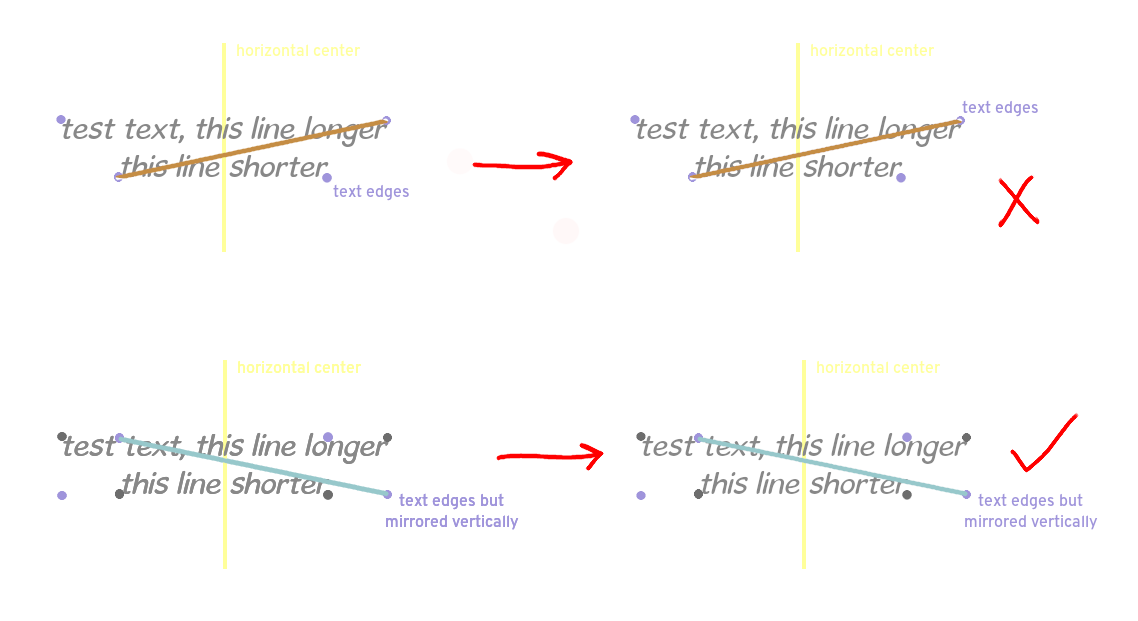We need to account for the fact that center of the ellipse is going to gravitate toward longer lines.

Once we have both coordinates of the center, we still have some leftover data from the matrix that we can use to determine both radii.

However, this approach doesn’t cover all the cases (it fails at least one), and the ‘offset corners by half a pixel’ way of dealing with things doesn’t seem to harm it, so we’ll just keep both in for the time being.

All in all, we’re progressing somewhat nicely. There’s some work to be done when determining the anti-jag parameter of the rectangular selection (but that — as well as padding — will be user-provided arguments/options). The only thing we have to deal with now are the bubbles with one or two lines, where our current tactics for determining the bubble size fails.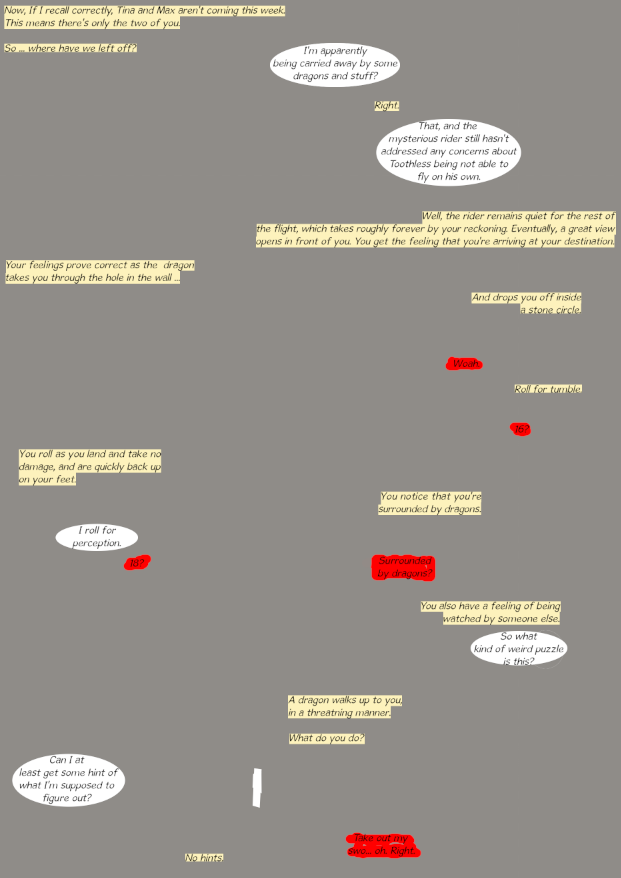Testing grounds. Things are mostly fine, except the bit where my script won’t handle the red bits.

Turns out that — spoiler alert — the brute force approach is the only approach that will consistently work. Maybe it should be revisited.

## How to make speech bubbles draw themselves: a story of pain (part 1)

Every now and then, I sit down in front of my computer and start making a comic. It follows a very similar premise to DM of the Rings and Darths&Droids — that is, take a movie (in my case, How To Train Your Dragon) and pretend it’s a D&D session — except my work is much less original and not that good, probably. Oh well.

If you’re making any sort of comics, you’ll probably have to draw a ton of speech bubbles. Mind, drawing basic speech bubbles isn’t that hard: you use the oval selection tool, select an area and fill it with color. But boy does it take time. The more of them, the longer it takes — and boy do some pages feature a lot of them.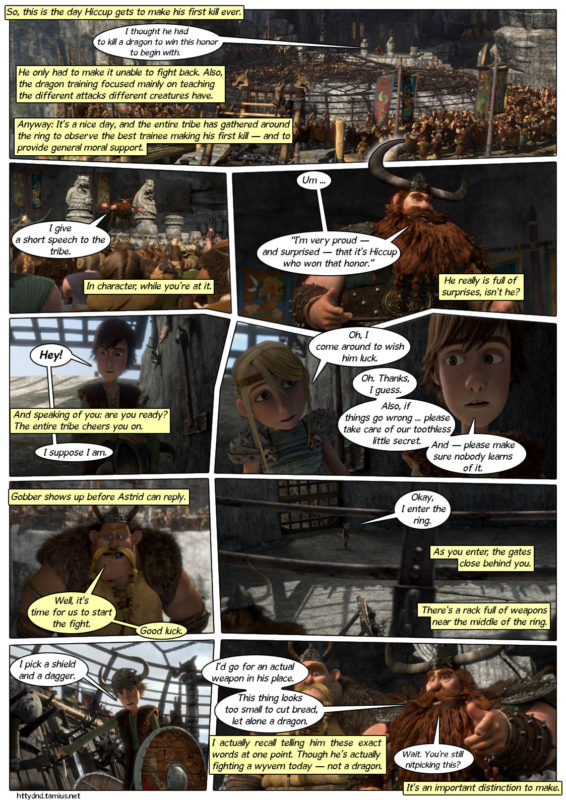Just to illustrate how bad it can get. Now admittedly an episode normally won’t have this many bubbles, but that doesn’t mean drawing speech bubbles isn’t a colossal waste of time.

Because we like to work smarter, not harder, the question pops up: why don’t we make a script that would draw speech bublbes for us? I’m using GIMP anyway, and GIMP has plugin support. ‘‘This shouldn’t be too hard,’’ were the famous last words as I opened visual studio code on that day about two weeks ago and got to work.

# Drawing rectangles is easy. Drawing ellipses is hard.

Comics often use two kinds of bubbles: there’s bubbles used for narration, which are often rectangular; and there’s bubbles used for speech, which are generally elliptical (more or less).

## Determining borders

If we want to draw any kind of bubble (be it rectangular or elliptical), we must first figure out where to place it. This is the easy part, because:

1. we use GIMP and put the text for each bubble on its own, separate, transparent layer. That’s one of the important assumptions that we’ll make (especially for elliptical bubbles): we don’t reuse text layers for more than one bubble, and the layer is always transparent except for text.
2. images and layers are, in general, grids of squares (pixels), where each square is colored with a different color. This allows us to borrow some tricks from Ultrawidify: we’ll check every pixel of every row, and every pixel of every column for the presence of non-transparent pixels.

If we keep track of where the first non-transparent pixel was found for every row and column, we can figure out where the text starts and where it ends. If rows of text don’t overlap each other, we get the bounds of every row of text in the layer. And when we know that a row of text starts at line 32, ends at line 64, starts at column 3 and ends at column 125. That gives us 4 corner points per line of text, and that’s enough data to draw a rectangle. The vertices are at 3, 32; 125, 32; 3, 64; 125, 64. The procedure is so simple it doesn’t even deserve its own heading (although there is a few minor, seemingly simple improvements you can make to that). At least compared to what’s about to come.

## Ellipses can honestly piss off

### Do we even know what we’re trying to do?

Yes, actually. We want to draw an ellipse around a set of points, where:

1. All the corner points must be inside or on the edge of said ellipse
2. Points must be as close to ellipse edge as possible

In order to draw an ellise, we need to know:

• width and height of the ellipse
• center of the ellipse (or more accurately, upper left corner¹, but we can get that from width and height of the ellipse)

¹In computer graphics, the coordinate system is flipped over horizontal axis compared to the coordinate system used most everywhere else.

because those are the parameters GIMP’s ellipse selection tool takes. We already know the points that represent the edges of text. What we need to do is to take those points (bunch of pairs of x,y coordinates) and somehow convert that to parameters of the ellipse that will satisfy the two rules outlined above.

Computers are very good at two things: logic and maths. This means we need to tell it how to calculate the center, width and height from those points. Because I don’t recall that lesson from my math classes, I went to every programmer’s best friend (stackoverflow) and discovered that — spoiler alert — this is either a) more or less impossible or b) requires diploma or master’s degree in maths.

I studied computer science. We had maths, but not that kind of maths.

### Time to cheat

First of all, we subtly change our second requirement. We don’t require that the corner points are as close to the edge of the ellipse anymore, instead we focus on trying to draw the smallest ellipse possible. It’s less than ideal, but we’ll have to live with that.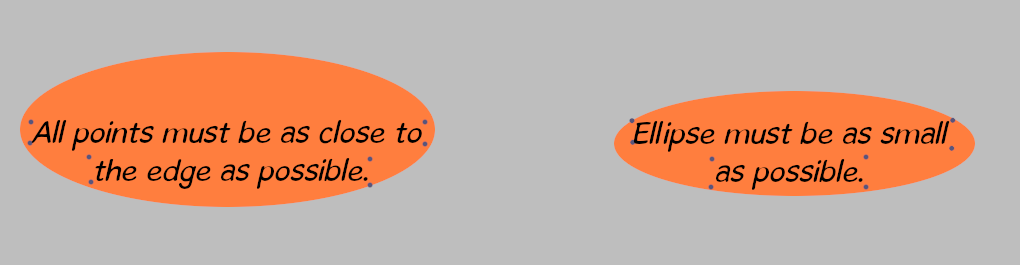It’s a subtle difference that only matters in edge cases like this. ‘Points as close to the edge as possible’ will give us a bigger ellipse overall, which is sometimes desired (if the upper portion of the speech bubble were to be covered with something). ‘Smallest ellipse’ produces lots of wasted space around the bottom line.Dots serve as a rough illustration of what the script would consider to be a corner point of the text.

After we’re done revising our requirements, we head back to google and look up the equations for the ellipse.

x = a * cos(t)
y = b * sin(t)

Where x, y are coordinates of a point on the ellipse; a, b are the length of the primary and secondary radii. We can do something with this. We can calculate where the center of ellipse will be. We just calculate where the middle point of every opposing pair of corner points is (e.g. the opposite point of upper left point in the top line of text is the lower right point in the bottom row of text). Since each pair of corner points may give a slightly different center, we take the average of those centers. Now that we know where the center is, we can use some high school maths to calculate the angle between straight line from center to a given point and the horizontal axis, and we do that for every point. Biggest ellipse will contain all the points, and we will have our answer.

Except t is not the angle to our point. tis the angle between horizontal axis and the point where our point would be, if the ellipse was squished (or stretched) into a circle. We can squish (or stretch) ellipse into a circle by multiplying (or dividing) one of the coordinates with the aspect ratio of the ellipse.

Do we know the aspect ratio of the ellipse? No.

We know the topmost point of the top line of text, we know bottommost point of the bottom line of text, we know leftmost and rightmost point of the longest line of text. We can get width and height from that. We can use that to calculate an aspect ratio.

Is that aspect ratio the same as the aspect ratio of the ellipse we want? Tests concluded that no. This is not the aspect ratio we’re looking for. Our final result looks something like this:White ellipse is what the script did. The selection has same dimensions than ellipse, but is placed roughly where the ellipse is supposed to be. Red lines represent what our script considers to be a line of text.Red line should be entirely within the ellipse (with at least 3 pixels to spare in the vertical direction, and 7 pixels to spare in horizontal). But the red seems to poke out for some reason.And not only does the ellipse fail to contain the lines completely, it’s also offset to the left and a little bit to the right.

Can we fix the aspect ratio with some brute force? I could write another paragraph on how this was attempted, but long story short: turns out we can’t. We’d need more data to draw the ellipse we want.

Oddshot with sound here.

Bummer. Turns out cheating doesn’t pay (yet). I guess we’ll have to do things the proper way™.